## Chapter 14: Exercises

Old Chinese version

1. (*) Median for minimizing L-1 norm: For a given vector x = [x1, ... xn], prove that the median of elements in x can minimize the following objective function based on L-1 norm:
Median of x = arg minu S|xi-u|
2. (*) Mean for minimizing L-2 norm: For a given vector x= [x1, ... xn], prove that the mean of elements in x can minimize the following objective function based on L-2 norm:
Mean of x = arg min u S(xi-u)2
3. (**) Function for for note segmentation: Write an m-file function myPv2note.m for note segmentation, with the following usage:
note = myPv2note(pitchVec, timeStep, pitchTh, minNoteDuration)
where
• pitchVec: The input pitch vector
• timeStep: The time for each pitch point
• pitchTh: Pitch threshold for creating a new note
• minNoteDuration: The minimum duration of a note
• note: The output note vector of the format [pitch, duration, pitch, duration, ...], where the units for pitch and duration are semitone and second, respectively.
Besides using pitch difference, you also need to use rests (zeros in pitchVec) for note segmentation. The duration of a rest note can be shorter than minNoteDuration. How to test your function:
1. Please use the pv file of "Happy Birthday" for note segmentation. You can hear the original wave clip and the corresponding midi file.
2. Please use the pv file of "Twinkle Twinkle Little Star" for note segmentation. You can hear the original wave clip and the corresponding midi file.
Hint:
• You can start with the pv2note.m in the SAP Toolbox, which do not take rests into consideration.
• You can use pvPlay.m to play the pitch vector, and use notePlay.m to play the output notes.
4. (**) Function for linear scaling: Pleae write an m-file function mylinearScaling4qbsh.m for linear scaling, with the following usage:
[minDist, bestPitch, allDist] = myLinearScaling4qbsh(queryPitch, dbPitch, lowerRatio, upperRatio, resolution, distanceType)
where
• queryPitch: the pitch vector of the input query. This is the vector to be expanded or contracted.
• dbPitch: a pitch vector in the song database
• lowerRatio: lower ratio for scaling
• upperRatio: upper ratio for scaling
• resolution: total number of scaling (contraction or expansion)
• distanceType: distnace type, 1 for L1 norm, 2 for L2 norm.
• minDist: mininum distance of the linear scaling
• bestPitch: the pitch vector of the input query after scaling (expansion or contraction) and transposition that can achieve the mininum distance
• allDist: all distances of linear scaling
Note that:
• If the expanded queryPitch is greater than the length of dbPitch, then stop doing linear scaling.
• For L-1 norm, we need to do median justification. For L-2 norm, we need to do mean justification.
• You need to perform distance normalization.
• You can assume the input vectors do not contain rests.
• Please run this example, but replace linScalingMex with myLinearScaling4qbsh to see if you can get the same result. (Note that in order to save computation, "linScalingMex" does not take the squar root when computing L-2 norm.)
• Use your function in the programming contest of QBSH to see if you can achieve the same recognition rate.
Here is the pseudo code for linear scaling: ... sf=linspace(lowerRatio, upperRatio, resolution); queryLen=length(queryPitch); for i=1:resolution Find the length of the scaled query (scaledQuery) Get out of the loop if scaledQuery is longer than dbPitch Obtain scaledQuery by interp1 Find the difference (diffPitch) between scaledQuery and dbPitch Find the median of diffPitch allDist(i)=norm(diffPitch-median, distanceType)/length(scaledQuery); scaledTransposedQuery{i}=... end [minDist, minIndex]=min(allDist); bestPitch=scaledTransposedQuery{minIndex}; ... Hint: You can use "interp1" for interpolation, "median" for computing median, "mean" for computing mean, "norm" for computing Lp norm. If you do not know how to use "interp1", the following is an example:

Example 1: interpDemo01.mn=10; x=1:n; y=rand(1,n); % Original vector (原始向量) ratio=1.75; % The vector interpolation should have a length equal to 1.8 times the original vector m=round(n*ratio); % Length of the scaled vector x2=linspace(1, n, m); y2=interp1(x, y, x2); % Vector after interpolation subplot(2,1,1), plot(x, y, 'o-', x2, y2, 'o:'); legend('Original vector', 'Interpolated vector', 'location', 'northOutside', 'orientation', 'horizontal'); subplot(2,1,2), plot(1:length(y), y, 'o-', 1:length(y2), y2, 'o:'); legend('Original vector', 'Scaled vector', 'location', 'northOutside', 'orientation', 'horizontal'); fprintf('Desired length ratio = %f\n', ratio); fprintf('Actual length ratio = %f\n', length(y2)/length(y));Desired length ratio = 1.750000 Actual length ratio = 1.800000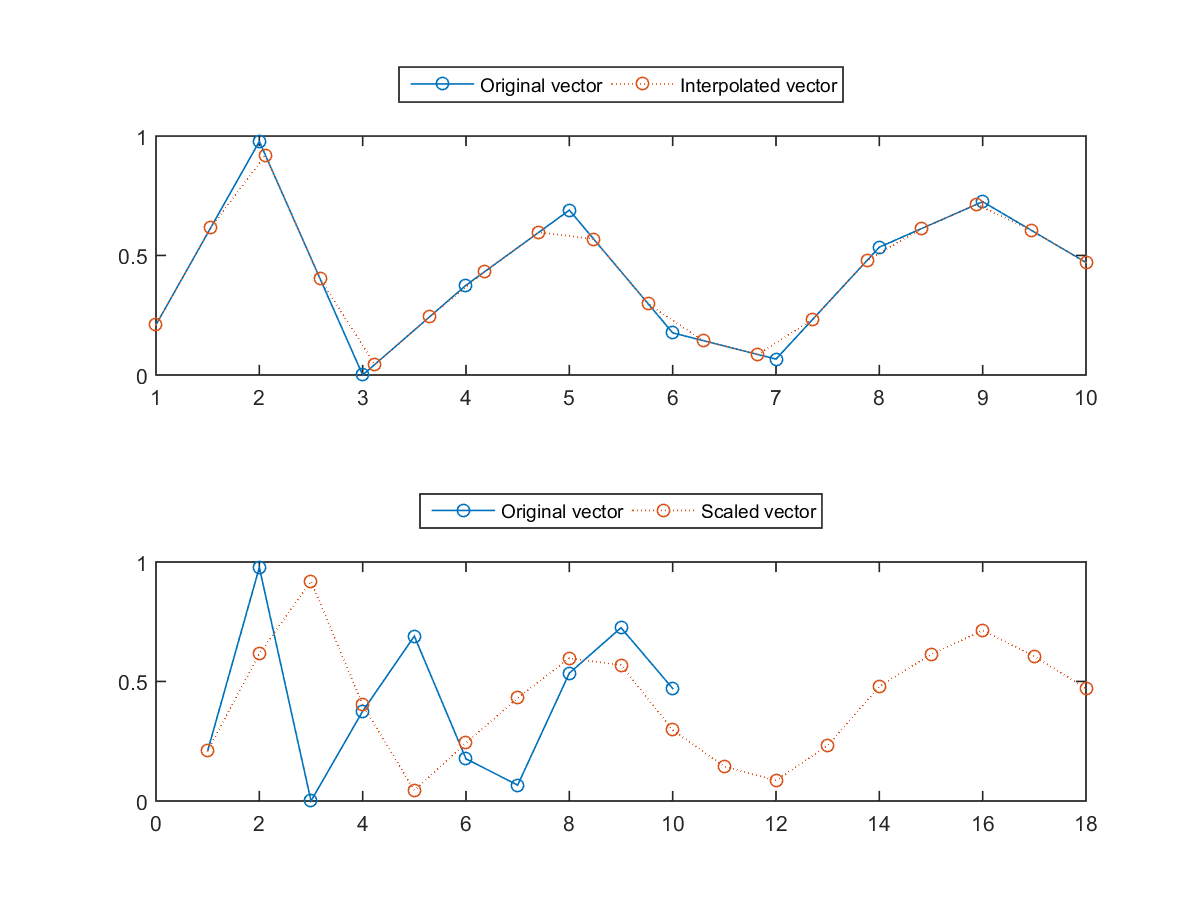5. (***) Linear scaling for chart detection: In this exercise, you need to write a function for chart detection in technical analysis of FinTech. Your function should look like this:
[distance, queryTransformed]=linScaling4chart(query, dbVec, sfMin, sfMax, sfCount, alphaMin);
where
• inputs:
• query: Chart pattern to be detected
• dbVec: Vector in the database, where the given query is to be found
• sfMin: Min. of the scaling factor
• sfMax: Max. of the scaling factor
• sfCount: Count of the scaling factors (which is equal to the no. of contractions/expansions of the query)
• alphaMin: Min. of alpha (Please check out the code template for more details.)
• outputs:
• distance: Normalized distance
• queryTransformed: The best transformed query (which is as close as possible to a portion in dbVec)
The steps of this function can be found in the following code template:

/linScaling4chartTemplate.mfunction [distMin, queryTransformedBest]=linScaling4chart(query, dbVec, sfMin, sfMax, sfCount, alphaMin) % linScaling: Linear scaling for chart detection % % Usage: % [distMin, queryTransformedBest, distAll]=linScaling4chart(query, dbVec, sfMin, sfMax, sfCount, alphaMin) sf=linspace(sfMin, sfMax, sfCount); % scaling factors queryLen=length(query); distAll=inf*ones(1, sfCount); queryTransformedAll=cell(1, sfCount); alphaAll=zeros(1, sfCount); for i=1:sfCount queryScaledLen=round(queryLen*sf(i)); % Length of the length-scaled query if queryScaledLen&gt;length(dbVec), break; end % Break if the length is too long queryScaled=... % Length-scaled query (compressed or expanded) seg=dbVec(1:queryScaledLen); % A segmeng of dbVec for comparison queryMean=mean(queryScaled); % Mean of query segMean=mean(seg); % Mean of seg queryJustified=queryScaled(:)-queryMean; % Zero-justified query segJustified=seg(:)-segMean; % Zero-justified seg alpha=... % Find alpha such that ||queryJustified*alpha-segJustified|| is minimized diff=queryJustified*alpha-segJustified; % Difference between queryJustified*alpha-segJustified rmse=... % root mean squared error distAll(i)=rmse/abs(alpha); % Normalized RMSE if alpha&lt;alphaMin, distAll(i)=inf; end % Set the distance to infinite if alpha is too small queryTransformedAll{i}=... % Record the transformed query alphaAll(i)=alpha; % Record alpha end [distMin, minIndex]=min(distAll); % Find the min distance queryTransformedBest=queryTransformedAll{minIndex}; % Find the best transformed query alphaBest=alphaAll(minIndex); % Find the best alpha

And here is a test example:

Example 2: linScaling4chart01.maddpath d:/users/jang/matlab/toolbox/utility % Utility Toolbox is available at http://mirlab.org/jang/matlab/toolbox/utility clear all; close all; %% Define the query (or chart, or pattern) to be detected T=20; t=(0:2*T-1)'; chartW=cos(2*pi*(1/T)*t); % Chart of "W" chartM=cos(2*pi*(1/T)*(t-T/2)); % Chart of "M" figure; plot(t, [chartW, chartM], 'marker', '.'); legend({'W', 'M'}, 'location', 'northOutside', 'orientation', 'horizontal'); %% Define variables and parameters query=chartW; dbVec=[74.4803 74.2668 74.9943 75.9871 75.1369 75.6966 73.8111 74.9851 74.6717 74.29 75.25 75.0641 74.4171 73.2131 74.3657 74.1497 74.2871 74.3763 75.4514 74.9987 74.0899 74.2214 73.5714 74.448 74.2571 74.82 76.2 77.9943 79.4386 78.7471 80.9031 80.7143 81.1287 80.0029 80.9186 80.7929 80.1943 80.0771 79.2043 79.6429 79.2843 78.6814 77.78 78.4314 81.4414 81.0957 80.5571 80.0129 79.2171 80.1457 79.0186 77.2829 77.7043 77.1482 77.6371 76.6456 76.1343 76.5329 78.0557 79.6229 79.1786 77.2386 78.4386 78.7871 79.4543 78.01 78.6429 72.3571 71.5357 71.3974 71.5143 71.6471 72.6843 73.2271 73.2157 74.24 75.57 76.5657 76.56 77.7757 77.7129 77.9986 76.7671 75.8786 75.0357 75.3643 74.58 73.9071 75.3814 75.1771 75.3943 75.8914 76.0514 75.8214 75.7771 75.8457 76.5843 76.6586 75.8071 74.9557 75.2486 75.9143 75.8943 75.5286 76.1243 77.0271 77.8557 77.1114 76.78 76.6943 76.6771 77.3786 77.5071 76.97 75.9743 74.7814 74.7771 75.76 74.7829 74.23 74.5257 73.9943 74.1443 74.9914 75.8814 75.957 74.9643 81.11 81.7057 84.87 84.6186 84.2986 84.4971 84.6543 85.8514 84.9157 84.6186 83.9986 83.6489 84.69 84.8229 84.8386 84.1171 85.3586 86.37 86.3871 86.6157 86.7529 87.7329 89.3757 89.1443 90.7686 90.4286 89.8071 91.0771 92.1171 92.4786 92.2243 93.7 94.25 93.86 92.29 91.28 92.2 92.08 92.18 91.86 90.91 90.83 90.28 90.36 90.9 91.98 92.93 93.52 93.48 94.03 95.968 95.35 95.39 95.035 95.22 96.45 95.32 94.78 93.0899 94.43 93.939 94.72 97.19 97.03 97.671 99.02 98.38 98.15 95.6 96.13 95.59 95.12 94.96 94.48 94.74 95.99 95.97 97.24 97.5 97.98 99.16 100.53 100.57 100.58 101.32 101.54 100.889 102.13 102.25 102.5 103.3 98.94 98.12 98.97 98.36 97.99 101 101.43 101.66 101.63 100.86 101.58 101.79 100.96 101.06 102.64 101.75 97.87 100.75 100.11 100.75 99.18 99.9 99.62 99.62 98.75 100.8 101.02 100.73 99.81 98.75 97.54 96.26 97.67 99.76 102.47 102.99 104.83 105.22 105.11 106.74 107.34 106.98 108 109.4 108.6 108.86 108.7 109.01 108.83 109.7 111.25 112.82 114.18 113.99 115.47 114.67 116.31 116.47 118.625 117.6 119 118.93 115.07 114.63 115.93 115.49 115 112.4 114.12 111.95 111.62 109.73 108.225 106.745 109.41 112.65 111.78 112.94 112.54 112.01 113.99 113.91 112.52]; sfMin=0.5; % Min of scaling factor sfMax=1.5; % Max of scaling factor sfCount=51; % Count of scaling factors minAlpha=0.0; % Minimum value of alpha %% distance=zeros(length(dbVec), 1); startPos=zeros(length(dbVec), 1); queryTransformed=cell(length(dbVec), 1); theDbLen=round(length(query)*sfMax); for i=1:length(dbVec) % fprintf('%d/%d\n', i, length(dbVec)); theDbVec=dbVec(i:min(i+theDbLen-1, length(dbVec))); [distance(i), queryTransformed{i}]=linScaling4chart(query, theDbVec, sfMin, sfMax, sfCount, minAlpha); startPos(i)=i; end localMinIndex=find(localMin(distance)); distance2=distance(localMinIndex); [~, sortIndex]=sort(distance2); fprintf('distance=%s\n', mat2str(distance)); n=10; % No of transformed queries to be plotted subplot(211); plot(dbVec) for i=1:n j=localMinIndex(sortIndex(i)); xIndex=startPos(j):startPos(j)+length(queryTransformed{j})-1; line(xIndex, queryTransformed{j}, 'color', getColor(i), 'lineWidth', 2); end line(1:length(dbVec), dbVec, 'color', 'k'); title('dbVec and identified charts'); subplot(212); localMin(distance, 1); set(gca, 'yscale', 'log'); for i=1:n j=localMinIndex(sortIndex(i)); line(j, distance(j), 'color', 'k', 'marker', '*'); end xlabel('Data index'); title('Distance and local minima'); distance=[2.37476192914899;2.05862449801402;1.69192516918267;1.56778216543534;1.65746536090226;1.60691672130988;1.65351096346274;1.17233541818172;1.04039952866266;1.25341625909422;2.0267928604897;2.69711715785997;2.61152637386103;2.51480515738034;2.22821315654657;2.14852543190902;2.14115541829409;2.21421262267844;2.49073525473915;3.5438324195636;6.18188134523823;15.1359868474017;Inf;13.34126269125;6.14128166023068;4.08362870062284;3.04106405867695;2.262410256329;1.67336110443637;1.4477214708692;1.20585386387712;1.0929951154194;0.960048608908948;0.898838229665287;0.859005880909992;0.940954192465787;1.14709144428376;1.52091699972465;1.53371975389737;1.39295325829491;1.28384433941832;1.17256815238096;1.06239554618634;0.930867547275527;0.853744044825693;0.833622875514638;0.848128644564756;0.880976422700091;0.935407030281707;1.01154523919148;1.19666213035879;1.43135171297128;1.67690948714386;1.86298380737413;1.63420728783791;1.48653784620003;1.28647539801525;1.11600568237351;1.0086778389479;0.832825453110207;0.711514441399075;0.622491809342115;0.571847427682915;0.58269271229184;0.652973578898635;0.835884870775472;1.20310303997524;2.17946124063812;2.93161106107837;3.6927241674757;4.99376539420183;3.2136287245863;2.06403228058992;1.65232275569824;1.44813682728363;1.27374446440195;1.15060078080548;1.09894490975872;0.889392009599149;0.699675569329099;0.671667994534988;0.717552279984247;0.910607503892355;1.31663994949726;1.41629764826173;1.38011215536551;1.36191967620249;1.28123115056617;1.10311417893135;1.05353455149711;0.981810139002471;0.952530405597213;0.974484038784851;1.03408174230056;1.10053190206141;1.20514173046717;1.36593682540791;1.68040435772177;2.15435568533838;2.4933814642994;2.61515996467349;2.72181701190362;2.89540540461785;2.94406519364514;2.74446317782029;2.66032551681612;2.73942989440114;2.65200013282373;2.52205553915111;2.37865296990994;2.3009554936854;2.27497452328198;2.38874855919574;2.54888866617287;2.8033941717755;3.06752193890088;3.17196171388327;3.4570868870802;4.38833775428644;5.97114944097118;7.84145065926738;17.0277984543384;Inf;Inf;16.4275279440298;8.30088071429423;5.14049219376976;3.29398287579397;2.87778876006141;2.56981883510195;2.58128489320369;2.59861028061893;2.5955131188562;2.65533408573774;2.78954242357178;3.17301969372607;3.49911455170319;3.83424659228042;4.10948244129052;4.22854560606094;4.64823850293707;5.27665108045275;5.7999398683492;5.50739717166017;6.00731123018192;7.61992245644834;8.24427938906146;7.99757144069162;6.29250122624244;6.00853768095942;8.17175916307257;4.28758912037837;3.21143811170636;2.65099520683645;2.32099407982487;2.17391494851049;2.15189441781247;1.80192106716997;1.54563324557573;1.50026461237139;1.53425361241134;1.60790298550378;1.57974811872238;1.49101025071401;1.60845589500979;1.85812405448433;2.37854218046262;3.29148130567015;4.43110014201054;6.27104780268827;4.6753534731494;3.21812481439331;2.24761530652258;1.67190670385145;1.3328588760048;1.06866586040143;0.807058065100112;0.631186364190041;0.621428329490589;0.615199772584618;0.650354835455285;0.729361500822739;0.963921485463435;1.74550862437733;4.06142531747234;3.75494426189952;2.55983663199755;2.00820395176405;1.58902484982958;1.32522049824415;1.28876116856777;1.24432784200853;1.27271597059454;1.46609049596584;1.74868505717001;2.17713232126561;2.43567404582421;2.97436812822285;3.61052153705257;4.14558400466692;4.46400716624194;4.08848363367285;3.48177073579111;2.94748850693566;2.34391727058525;1.97167798262777;1.6741529043574;1.45043010790681;1.3200317061345;1.29423682937752;1.28410796972362;1.10855689441525;0.988906863241172;0.952823158701207;0.973209123914305;1.2065344805565;1.61504970882742;3.06243129511788;5.75522298112339;5.6160099664048;4.41200881510493;3.58234415727809;2.70409544058045;1.93047024255975;1.75514991146223;1.62745225502015;1.48363351185616;1.34674365767221;1.17604456588497;1.09615273536985;1.00586919293912;0.913630239807206;0.886389468927065;0.980548607715331;0.976186723814148;0.848242700328003;1.71917406131292;5.03014562970379;4.69357502039522;4.12054810762944;3.66728157776704;3.35388804088922;3.24759423777433;3.09950272210888;3.54910375831611;4.95619694327707;11.2225115459901;11.1378331478593;8.67096579549509;6.16641808353592;4.16980362797127;3.07992781093481;2.48483281158353;2.22655188492873;2.02406333199073;1.96762800535378;1.91961541157564;1.87568541197916;1.96882709378323;2.18546255906645;2.3868615181307;2.78212403666092;3.78279005284333;5.04707802158397;7.0716999863914;9.28494128695091;11.0902571373853;9.68056756616905;8.0653796216565;7.52130423876936;7.5520502498099;7.72860228424887;5.96782238110945;4.8601087259383;3.20907527765714;2.44228788428646;1.95682789170052;2.32842712956508;4.97597569963609;Inf;Inf;Inf;Inf;Inf;Inf;Inf;Inf;Inf;Inf;Inf;Inf;Inf;Inf;Inf;Inf;Inf;Inf;Inf;Inf;Inf;Inf]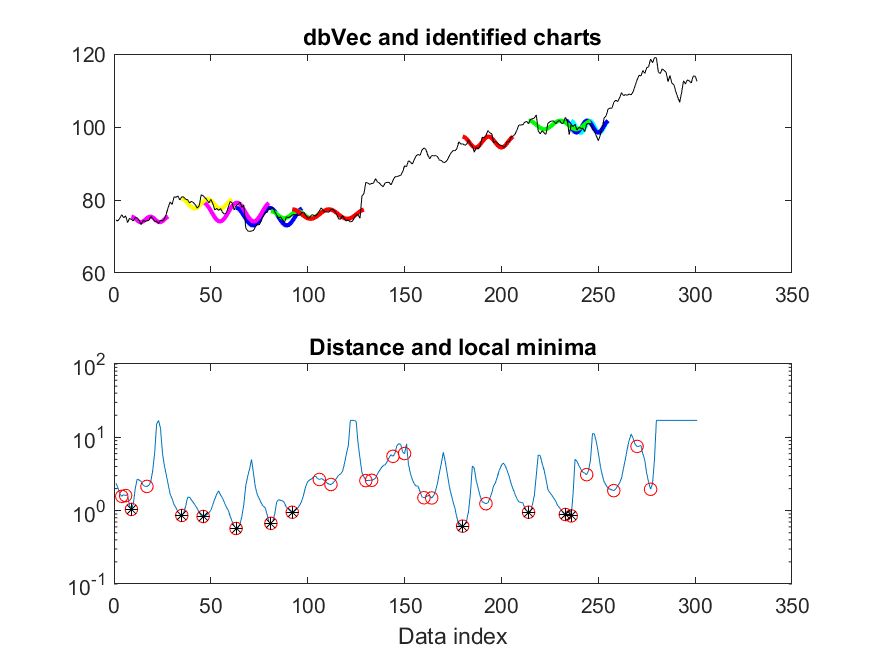6. (***) Function for type-3 DTW: Please write a m-file function myDtw3.m for type-3 DTW, with the usage:
[minDist, dtwPath, dtwTable] = myDtw3(vec1, vec2)
You can start from the following skeleton:

原始檔（dtw3skeleton.m）：（灰色區域按兩下即可拷貝）
function [minDistance, dtwPath, dtwTable]=dtw3(vec1, vec2, beginCorner, endCorner)
% dtw3: Dynamic time warping with local paths of 0 and 45 degrees
%	Usage: [minDistance, dtwPath, dtwTable]=dtw3(vec1, vec2, beginCorner, endCorner, plotOpt)
%		vec1: testing vector, which should be a pitch vector
%		vec2: reference vector, which should be a vector of note pitch
%		minDistance: minimun distance of DTW
%		dtwPath: optimal path of dynamical programming through the DTW table
%			(Its size is 2xk, where k is the path length.)
%		dtwTable: DTW table

if nargin<3, beginCorner=1; end
if nargin<4, endCorner=1; end

% If input is vector, make it row vector
if size(vec1,1)==1 | size(vec1,2)==1, vec1 = vec1(:)'; end
if size(vec2,1)==1 | size(vec2,2)==1, vec2 = vec2(:)'; end

size1=length(vec1);
size2=length(vec2);

% ====== Construct DTW table
dtwTable=inf*ones(size1,size2);
% ====== Construct the first element of the DTW table
dtwTable(1,1)=vecDist(vec1(:,1), vec2(:,1));
% ====== Construct the first row of the DTW table (xy view)
for i=2:size1
dtwTable(i,1)=dtwTable(i-1,1)+vecDist(vec1(:,i), vec2(:,1));
prevPos(i,1).i=i-1;
prevPos(i,1).j=1;
end
% ====== Construct the first column of the DTW table (xy view)
if beginCorner==0
for j=2:size2
dtwTable(1,j)=vecDist(vec1(:,1), vec2(:,j));
prevPos(1,j).i=[];
prevPos(1,j).j=[];
end
end

% ====== Construct all the other rows of DTW table
for i=2:size1
for j=2:size2
pointDist=vecDist(vec1(:,i), vec2(:,j));
% ====== Check 45-degree predecessor
...
% ====== Check 0-degree predecessor
...
end
end

% ====== Find the overall optimum path
[minDistance, dtwPath]=dtwBackTrack(dtwTable, prevPos, beginCorner, endCorner);

% ========== Sub function ===========
function distance=vecDist(x, y)
distance=sum(abs(x-y));

1. Try this example, but replace dtw3mex by myDtw3 to see if you can obtain the same result.
2. Use your function in the programming contest of QBSH, with 1 key transposition. What is the recognition rate?
3. Use your function in the programming contest of QBSH, with 5 key transpositions. What is the recognition rate?
7. (***) Methods for improving type-3 DTW: Write a m-file function myDtw3b.m for type-3 DTW, with the enhancements mentioned at the end of the section covering type-3 DTW:
1. Set the range of frame numbers being mapped to each note: For instance, the duration of the frames being mapped to a note should between the range [0.5, 2] of the duration of the note. This can enhance the performance since the duration of each note is used.
2. Use rests: Except for the leading and trailing rests, any rest in the query input indicates the end of the previous note and the beginning of the next note. We can use this cue for better alignment and query by singing/humming.
3. Use both of the above enhancements.
1. Use your function in the programming contest of QBSH, with L-1 norm and 1 key transposition. What is the three recognition rates?
2. Use your function in the programming contest of QBSH, with L-1 norm and 5 key transpositions. What is the three recognition rates?
8. (***) QBSH accuracy w.r.t. LS resolutions and no. of key transpositions: Before attempting this exercise, you should first fully understand the example program in the programming contest of QBSH. You tasks are explained next.
1. Use linear scaling for QBSH, and plot the recognition rate as a function of the computation time, but parameterized by the resolution for linear scaling from 5 to 12. Your plot should be similar to the following one: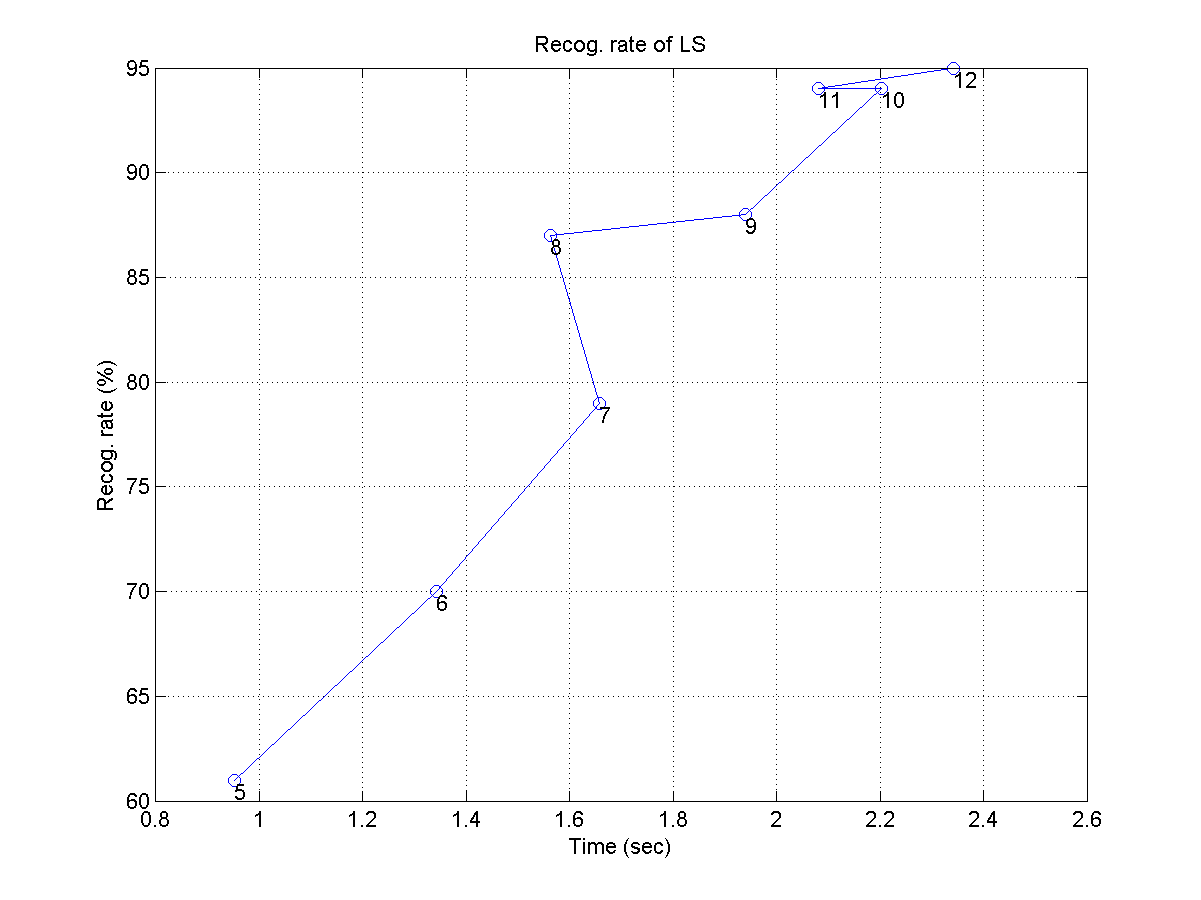2. Use type-1 DTW for QBSH, and plot the recognition rate as a function of the computation time, but parameterized by the numbers of key transposition of [1, 3, 5, 7, 9]. Your plot should be similar to the following one: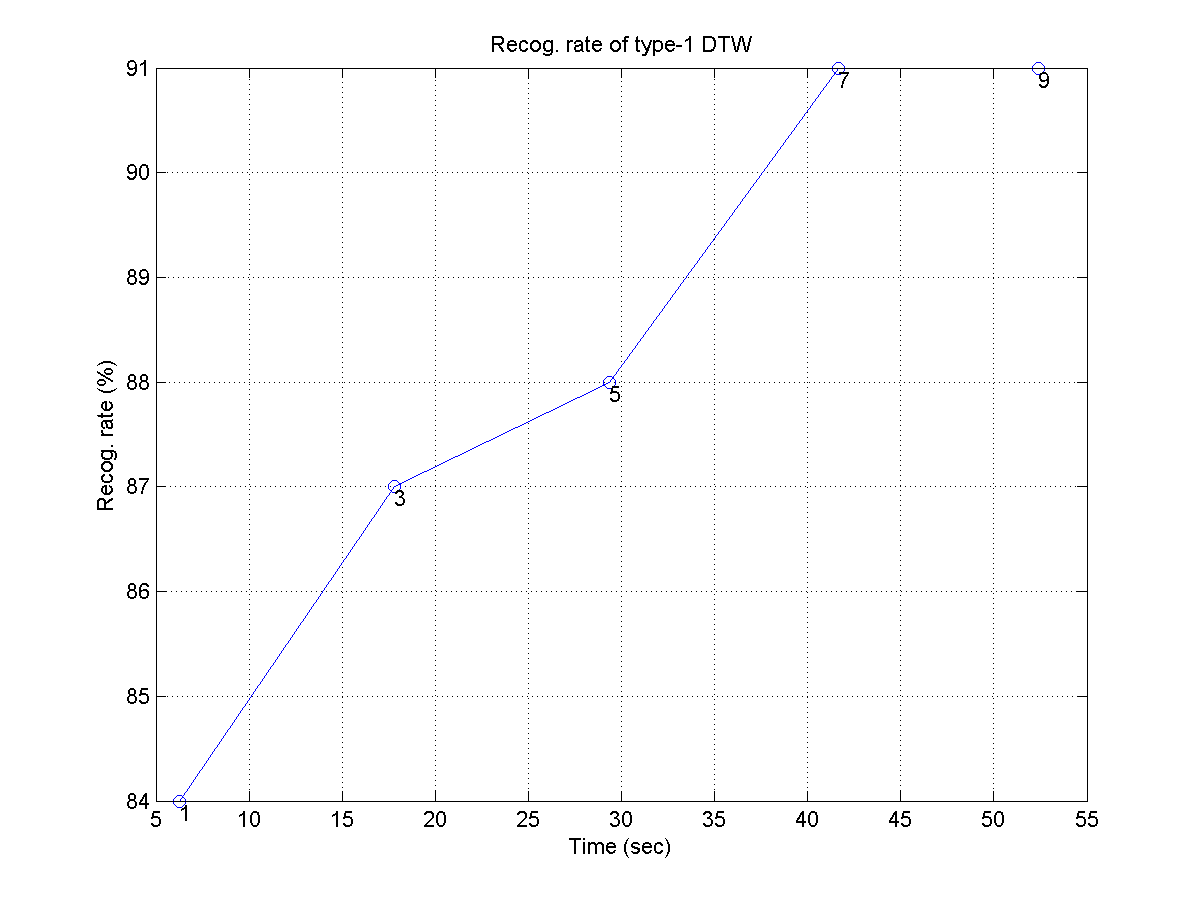Hint:
• If the computing time is too long, you can use the first 100 wave files for this exercise.
• It would be easier for you to do the computation and plotting if you modify the example program in the programming contest for QBSH into a single function.
9. (***) QBSH accuracy w.r.t. pitch vector reduction ratio: Before attempting this exercise, you should first fully understand the example program in the programming contest of QBSH. The pitch rate of our query pitch vector is fs/frameSize = 8000/256 = 31.25. If the computing time is too long, we can simply down-sample the pitch vector to reduce the size of the DTW table. In other words, we can explore the effect of PVRR (pitch vector reduction ratio) on the recognition rates of various methods. For a given value of pvrr, the pitch rate becomes 31.25/pvrr. You need to plot the recognition rates of 4 methods (LS, DTW1, DTW3, DTW3) with respect to computation time, but parameterized by PVRR which varies from 1 to 10. Your plot should be similar with the following one: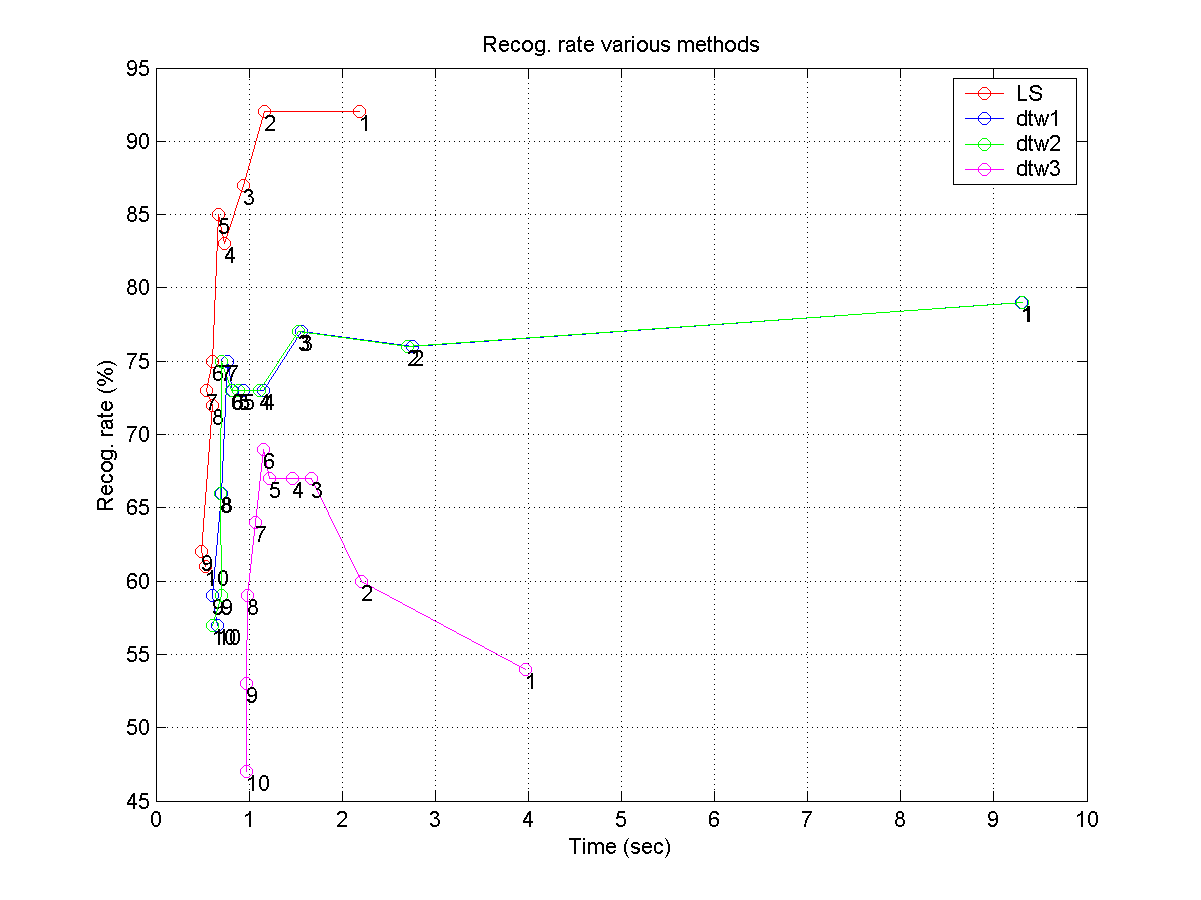Hint:
• If the computing time is too long, you can use the first 100 wave files for this exercise.
• It would be easier for you to do the computation and plotting if you modify the example program in the programming contest for QBSH into a single function.
10. (***) Creating a QBSH prototype using linear scaling: In this exercise, you are going to write a MATLAB script to create a prototypical QBSH system. Basically, the user can sing/hum to the microphone for 8 seconds and the system can list the top-10 ranking of the retrieved songs based on their similarity to the query input. Before trying this exercise, you should download the following toolboxes and add them to your search path: Your system should follow the following steps:
1. Load the song database. This can be achieved by using
You can check the contents of songDb by typing
structDispInHtml(songDb);
In particular, songDb(i).track is a vector in the format of [pitch1, duration1, pitch2, duration2, ...], which specifies the melody track of the i-th song in the database, where pitch is in the unit of semitones and duration is in the unit of 1/64 seconds.

Once you have loaded the songs into memory, you can play the songs by using notePlay. For instance, if you want to play the first 30 notes of "Yankee Doodle", try the following command:

notePlay(songDb(42).track(1:60), 1/64);
(Remember to turn on your speakers.) If you want to see the pitch contour of the song, try
notePlot(songDb(42).track, 1/64);
If you want to see the pitch contour together with the generated waveform, try:
notePlay(songDb(42).track(1:60), 1/64, 1, 1)
2. Convert the "track" field of each song to PV (pitch vector) representation and attach the PV to a field "pv" of songDb. You can use the command note2pv. For instance:
[pv, noteStartPvIndex]=note2pv(songDb(42).track, 1/31.25, [], inf, 1);
The above command will convert song 42 (Yankee Doodle) into a pitch vector pv, with a pitch rate of 31.25. A plot will also be shown to demonstrate how the music notes are converted into PV. (Please try "help note2pv" for detailed usage.)
3. Do 8-second recording with fs=8000, nbits=8.
4. Perform pitch tracking by using the command ptByPf. Plot the result of pitch tracking (by supplying appropriate arguments to the command) after each recording to make sure the pitch tracking result is satisfactory. (Try "help ptByPf" for its usage and example.)
5. Handle rests by using the command restHandle.
6. Compare the query PV to each PV in the database using linScalingMex. (Please make sure you understand the example of linear scaling used in the text.)
7. Display the top-10 retrieved songs based on the LS distances.
You system should be able to let the user sing/hum as many songs as they want until ctrl-c is hit. When you demo your system to TA, make sure you can have at least 3 humming/singing inputs to have the correct answer in top-10 results.
11. (***) Creating a QBSH prototype using DTW: Repeat the above exercise using DTW. Make sure to explain to the TA how you perform key transposition.